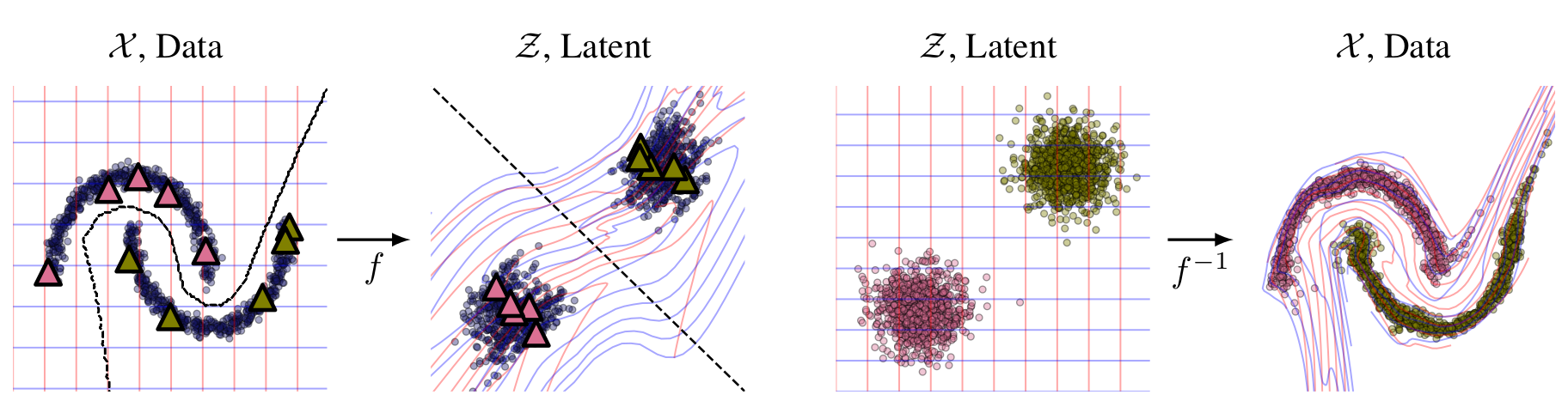# izmailovpavel / flowgmm

No description, website, or topics provided.
Jupyter Notebook Shell Python
Latest commit aee5a19 Dec 30, 2019
Type Name Latest commit message Commit time
Failed to load latest commit information.data Dec 29, 2019experiments Dec 30, 2019flow_ssl Dec 29, 2019README.md Dec 30, 2019

# Flow Gaussian Mixture Model (FlowGMM)

This repository contains a PyTorch implementation of the Flow Gaussian Mixture Model (FlowGMM) model from our paper

Semi-Supervised Learning with Normalizing Flows

by Pavel Izmailov, Polina Kirichenko, Marc Finzi and Andrew Gordon Wilson.

# Introduction

Normalizing flows transform a latent distribution through an invertible neural network for a flexible and pleasingly simple approach to generative modelling, while preserving an exact likelihood. In this paper, we introduce FlowGMM (Flow Gaussian Mixture Model), an approach to semi-supervised learning with normalizing flows, by modelling the density in the latent space as a Gaussian mixture, with each mixture component corresponding to a class represented in the labelled data. FlowGMM is distinct in its simplicity, unified treatment of labelled and unlabelled data with an exact likelihood, interpretability, and broad applicability beyond image data.

We show promising results on a wide range of semi-supervised classification problems, including AG-News and Yahoo Answers text data, UCI tabular data, and image datasets (MNIST, CIFAR-10 and SVHN).# Usage

We provide the scripts and example commands to reproduce the experiments from the paper.

## Synthetic Datasets

The experiments on synthetic data are implemented in this ipython notebook. We additionaly provide another ipython notebook applying FlowGMM to labeled data only.

## Image Classification

To run experiments with FlowGMM on image classification problems you first need to download and prepare the data. To do so, run the following scripts:

```./data/bin/prepare_cifar10.sh
./data/bin/prepare_mnist.sh
./data/bin/prepare_svhn.sh```

To run FlowGMM, you can use the following script

```python3 experiments/train_flows/train_semisup_cons.py \
--dataset=<DATASET> \
--data_path=<DATAPATH> \
--label_path=<LABELPATH> \
--logdir=<LOGDIR> \
--ckptdir=<CKPTDIR> \
--save_freq=<SAVEFREQ> \
--num_epochs=<EPOCHS> \
--label_weight=<LABELWEIGHT> \
--consistency_weight=<CONSISTENCYWEIGHT> \
--consistency_rampup=<CONSISTENCYRAMPUP> \
--lr=<LR> \
--eval_freq=<EVALFREQ> \```

Parameters:

• `DATASET` — dataset name [MNIST/CIFAR10/SVHN]
• `DATAPATH` — path to the directory containing data; if you used the data preparation scripts, you can use e.g. `data/images/mnist` as `DATAPATH`
• `LABELPATH` — path to the label split generated by the data preparation scripts; this can be e.g. `data/labels/mnist/1000_balanced_labels/10.npz` or `data/labels/cifar10/1000_balanced_labels/10.txt`.
• `LOGDIR` — directory where tensorboard logs will be stored
• `CKPTDIR` — directory where checkpoints will be stored
• `SAVEFREQ` — frequency of saving checkpoints in epochs
• `EPOCHS` — number of training epochs (passes through labeled data)
• `LABELWEIGHT` — weight of cross-entropy loss term (default: `1.`)
• `CONSISTENCYWEIGHT` — weight of consistency loss term (default: `1.`)
• `CONSISTENCYRAMPUP` — length of consistency ramp-up period in epochs (default: `1`); consistency weight is linearly increasing from `0.` to `CONSISTENCYWEIGHT` in the first `CONSISTENCYRAMPUP` epochs of training
• `LR` — learning rate (default: `1e-3`)
• `EVALFREQ` — number of epochs between evaluation (default: `1`)

Examples:

```# MNIST, 100 labeled datapoints
python3 experiments/train_flows/train_semisup_cons.py --dataset=MNIST --data_path=data/images/mnist/ \
--label_path=data/labels/mnist/100_balanced_labels/10.npz --logdir=<LOGDIR> --ckptdir=<CKPTDIR> \
--save_freq=5000 --num_epochs=30001 --label_weight=3 --consistency_weight=1. --consistency_rampup=1000 \
--lr=1e-5 --eval_freq=100

# CIFAR-10, 4000 labeled datapoints
python3 experiments/train_flows/train_semisup_cons.py --dataset=CIFAR10 --data_path=data/images/cifar/cifar10/by-image/ \
--label_path=data/labels/cifar10/4000_balanced_labels/10.txt --logdir=<LOGDIR> --ckptdir=<CKPTDIR> \
--save_freq=500 --num_epochs=1501 --label_weight=3 --consistency_weight=1. --consistency_rampup=100 \
--lr=1e-4 --eval_freq=50```

## Tabular Data: UCI and NLP

For class balanced data splitting and for training of FlowGMM on the UCI and NLP datasets, we use the snake-oil-ml library.

### UCI Data Preparation

Downdload the miniboone and hepmass datasets here. We follow the preprocessing (where sensible) from Masked Autoregressive Flow for Density Estimation. Unpack the files into a reasonable location (the default expected location for the files are ~/datasets/UCI/hepmass/ and ~/datasets/UCI/miniboone/).

### NLP Data Preparation

To run experiments on the text data, you first need to download the data and compute the BERT embeddings. To get the data run `data/nlp_datasets/get_text_classification_data.sh`. Then, you this ipython notebook shows an example of computing BERT embeddings for the data.

### Running the Models

After the data has been prepared, the notebook here can be used to run the kNN, Logistic Regression, and Label Spreading baselines.

The 3-Layer NN with dropout and Pi-Model baseline experiments are implemented in train_semisup_text_baselines.py.

Finally the FlowGMM method can be trained on these datasets using train_semisup_flowgmm_tabular.py.

# References

You can’t perform that action at this time.